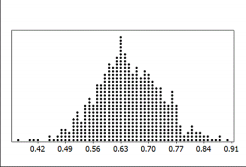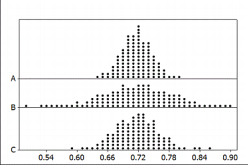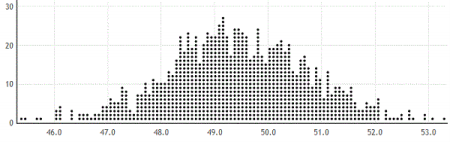×
Get Full Access to NAU - STA 270 - Study Guide - Midterm
Get Full Access to NAU - STA 270 - Study Guide - Midterm

×

NAU / Statistics / STAT 270 / Based on the goal to predict foot length from forearm length, which va

# Based on the goal to predict foot length from forearm length, which va Description

##### Description: Practice Exam 2
8 Pages 130 Views 2 Unlocks
Reviews

STA 270 Spring 2017 Exam 2 Study Guide

## Based on their goal to predict foot length from forearm length, which variable is the explanatory variable?For many sections, the test will cover Section 2.6 and Chapter 3 in your text. Please double check with your teacher to know which sections will be tested in your class.

You will want to bring a calculator.

You may use a 3” x 5” index card with notes on both sides.

Section 2.6 – Know how to differentiate between the explanatory and response variable, how to read the StatKey output to construct the least-squares regression line, and how to use the regression line to predict values for one variable given a value of the other variable, and compute a residual. Know how to interpret slope, intercept and residual. Know when it is appropriate to use the regression line.

Chapter 3 – the main skills in this chapter are constructing and interpreting confidence intervals. However, in order for you to do this properly, you also need to comfortable choosing the correct parameter and statistic for a given scenario.

## Which type of association positive, negative, close to 0 would you expect to be true about the association between the length of the forearm and the length of the foot?­­­­­­­­­­­­­­­­­­­­­­­­­­­­­­­­­­­­­­­­­­­­­­­­­­­­­­­­­­­­­­­­­­­­­­­­­­­­­­­­­­­­­­­­­­­­­­­­­­­­­­­­­­­­­­­­­­­­­­­­­­­­­­­­­­­­­­­­­­­­­­­­­­­­­­­

1. Students in a small statistics course collected data to determine if the length of the forearm could be used to predict the  length of the foot (both measured in centimeters). Their data are displayed in the provided table.

Forearm (cm)

29

28

27

23

26

29.5

36

29

30

24

27

29.5

32

Foot (cm)

26

23

24

23

25

27

29

28

23

23

24

26

31

## If it makes sense, interpret the slope of your regression line. if it does not, explain why not?If you want to learn more check out When was the national woman's party established?

(a)Based on their goal (to predict foot length from forearm length), which variable is the explanatory variable? (b)Which type of association (positive, negative, close to 0) would you expect to be true about the association between  the length of the forearm and the length of the foot?

(c) What does it mean for the two variables to have the association described above?

(d) Two outputs from Statkey for this data set are shown on the next page.  Use the correct output and find the least  squares regression equation for predicting foot length from forearm length. Don't forget about the age old question of Is a hypothesis an educated guess?

(e) If it makes sense, interpret the slope of your regression line.  If it does not, explain why not. (f) If it makes sense, interpret the intercept of your regression line.  If it does not, explain why not. (g) Use your regression line to predict the foot length of a person whose forearm measures 30 cm. (h) Use your regression line to predict the forearm length from a person whose foot measures 30 cm. (i) Use your regression line to predict the foot length of a person whose forearm measures 50 cm. (j) Use your regression line to predict the forearm length from a person whose foot measures 50 cm. (k) Compute the residual when the forearm length is 30 cm.

Page 1

Page 2

2. A random sample of 200 students shows that 62% of students use the Student Health Center at some point during their  ±

time on campus, with a margin of error of

4%. Based on this information, identify each of the following as plausible

or not for the percent of the entire student body that use the Student Health Center at some point during their time on  campus.

(a) 50% (b) 60% (c) 65% (d) 72% If you want to learn more check out How does the evolutionary perspective explain human behavior?

3.  Identify each of the following as either a parameter or a statistic, and give the correct notation.

(a) Correlation between height and arm span (distance from fingertip to fingertip when arms are extended to the sides)  for all players on the Chicago Bulls basketball team, using data from all players currently on the team (b) Proportion of students at your university that smoke, based on data from your class.

(c) Average commute time for employees at a small company, based on interviews with all employees. (d) Proportion of students at a university that are part­time, based on data on all students enrolled at the university.

4.  Briefly explain the distinction between a parameter and a statistic.

5.  Identify whether each of the following is a possible bootstrap sample from this original sample: 20, 24, 19, 23, 18

(a) 24, 19, 24, 20,23

(b) 20, 24, 21, 19, 18

(c) 18, 19, 20, 23, 24

Page 3

6.  The sampling distribution shows sample proportions from samples of size n = 35.Don't forget about the age old question of How do you introduce a role play in the bedroom?

(a) What does one dot on the sampling distribution represent?

(b) Estimate the population proportion from the dotplot.

(c) Estimate the standard error of the sample proportions.

(d) Using the sampling distribution, how likely is  (e) Using the sampling distribution, how likely is  (f) Using the sampling distribution, how likely is

= 0.65?

= 0.45?

= 0.98?

(g) If samples of size n = 65 had been used instead of n = 35, which of the following would be true? i. The sample statistics would be centered at a larger proportion.

ii. The sample statistics would be centered at roughly the same proportion.

iii. The sample statistics would be centered at a smaller proportion.

(h) If samples of size n = 65 had been used instead of n = 35, which of the following would be true? i. The sample statistics would have more variability. If you want to learn more check out What is the role of irb?

ii. The variability in the sample statistics would be about the same.

iii. The sample statistics would have less variability.

7.  In an August 2012 Gallup survey of 1,012 randomly selected U.S. adults (age 18 and over), 53% said that they were  dissatisfied with the quality of education students receive in kindergarten through grade 12. They also report that the  "margin of sampling error is 4%."

(a) What is the population of interest?

(b) What is the sample being used?

(c) What is the population parameter of interest, and what is the correct notation for this parameter? (d) What is the relevant statistic?

(e) Find an interval estimate for the parameter of interest. Interpret it in terms of dissatisfaction in the quality of  education students receive. Use two decimal places in your answer.

Page 4We also discuss several other topics like What are the five points about the nature of gender?

8.  According to U.S. Census data, 71.6% of Americans are age 21 and over. The provided figure shows possible sampling  distributions for the proportion of a sample age 21 and over, for samples of size n = 50, n = 125, and n = 250.Match the sample sizes (n = 50, n = 125, and n = 250) to their sampling distribution.

(a) Sample A:  n = _____________

(b) Sample B:  n = _____________

(c) Sample C:  n = _____________

9.  Suppose that a student collects pulse rates from a random sample of 200 students at her college and finds a 90%  confidence interval goes from 65.5 to 71.8 beats per minute. Is the following statement an appropriate interpretation of  this interval? If not, explain why not.

"90% of the students at my college have mean pulse rates between 65.5 and 71.8 beats per minute."

10. November 6, 2012 was Election Day. Many of the major television networks aired coverage of the incoming election  results during the primetime hours. The table below displays the amount of time (in minutes) spent watching election  coverage for a random sample of 25 U.S. adults.

123

120

45

30

40

86

36

52

86

2

70

155

70

168

156

107

126

66

71

97

73

90

69

5

68

Page 5

(a) What is the population parameter of interest? Define using the appropriate notation. (b) What is the sample statistic?  Define using the appropriate notation. (c) Using the appropriate formula, construct a 95% confidence interval for the parameter. (d) Using the percentile method, construct a 95% confidence interval for the parameter.

The bootstrap distribution you will need to answer c & d is on the top of the next page.   Page 6

Page 7

10. A 2009 study to investigate the dominant paws in cats was described in Animal Behavior (Volume 78, Issue 2). The researchers used a random sample of 42 domestic cats. In this study, each cat was shown a treat (5 grams of tuna), and while the cat watched, the food was placed inside a jar. The opening of the jar was small enough that the cat could not stick its head inside to remove the treat. The researcher recorded the paw that was first used by the cat to try to retrieve the treat. This was repeated 100 times for each cat (over a span of several days). The paw used most often was deemed the dominant paw (note that one cat used both paws equally and was classified as "ambidextrous"). Of the 42 cats studied, 20 were classified as "left­pawed".  The bootstrap distribution is on the bottom of the previous page.

(a) What is the population parameter of interest? Define using the appropriate notation.

(b) Describe how to use the data to construct a bootstrap distribution. What value should be recorded for each of the  bootstrap samples?

(c) Construct and interpret a 96.5% confidence interval based on the bootstrap distribution.

11. A bootstrap distribution, based on 1,000 bootstrap samples is provided. Use the distribution to estimate a 99% confidence interval for the population mean. Show/Explain how you arrived at your answer.12. Suppose we are interested in comparing the proportion of male students who smoke to the proportion of female students  who smoke. We have a random sample of 150 students (60 males and 90 females) that includes two variables: Smoke =  "yes" or "no" and Gender = "female (F)" or "male (M)". The two­way table below summarizes the results.

Smoke = Yes

Smoke = No

Sample Size

Gender = M

9

51

60

Gender = F

9

81

90

(a) Identify and describe the parameter of interest.

(b) Identify and give the value of the statistics from this study.

(c) Describe how to use the data to construct a bootstrap distribution. What value should be recorded for each of the  bootstrap samples?

(d) Use technology to construct a bootstrap distribution with at least 1,000 samples and estimate the point estimate and  standard error.

(e) Using the point estimate and standard error above, construct a 95% confidence interval for the difference in the  proportion of smokers between male and female students, pm ­ pf. Interpret the interval in the context of this data  situation.

(f) Use the bootstrap distribution to provide a 98% confidence interval for the difference in the proportion of smokers  between male and female students, pm ­ pf.

Page 8

Page ExpiredIt looks like your free minutes have expired! Lucky for you we have all the content you need, just sign up here